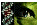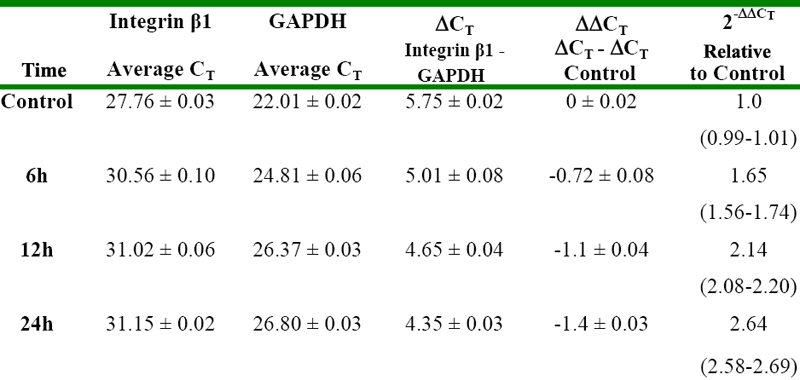Table 1 of Yao, Mol Vis 2007; 13:1769-1776.

Table 1. Relative quantitation using the comparative CT method A

The ΔCT value is determined by subtracting the average GAPDH CT value from the average integrin β1 CT value. The calculation of ΔΔCT involves subtraction by the ΔCT calibrator value. This is subtraction of an arbitrary constant, so the standard deviation of ΔΔCT is the same as the standard deviation of the ΔCT value. The range given for integrin β1 relative to the control is determined by evaluating the expression: 2-ΔΔCT with "ΔΔCT plus s" and "ΔΔCT minus s", where "s" equals the standard deviation of the ΔΔCT value.Yao, Mol Vis 2007; 13:1769-1776 <http://www.molvis.org/molvis/v13/a197/>
©2007 Molecular Vision <http://www.molvis.org/molvis/>
ISSN 1090-0535